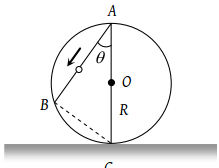Two bodies of different masses ma and mb are dropped from two different heights a and b. The ratio of the time taken by the two to cover these distances are

(1) a : b

(2) b : a

(3) $\sqrt{a}:\sqrt{b}$

(4) ${a}^{2}:{b}^{2}$

Concept Questions :-

Uniformly accelerated motion
High Yielding Test Series + Question Bank - NEET 2020

Difficulty Level:

A body falls freely from rest. It covers as much distance in the last second of its motion as covered in the first three seconds. The body has fallen for a time of

(1) 3 s

(2) 5 s

(3) 7 s

(4) 9 s

Concept Questions :-

Uniformly accelerated motion
High Yielding Test Series + Question Bank - NEET 2020

Difficulty Level:

A stone is dropped into water from a bridge 44.1 m above the water. Another stone is thrown vertically downward 1 sec later. Both strike the water simultaneously. What was the initial speed of the second stone ?

(1) 12.25 m/s

(2) 14.75 m/s

(3) 16.23 m/s

(4) 17.15 m/s

Concept Questions :-

Uniformly accelerated motion
High Yielding Test Series + Question Bank - NEET 2020

Difficulty Level:

A body is thrown vertically upwards. If air resistance is to be taken into account, then the time during which the body rises is

(1) Equal to the time of fall

(2) Less than the time of fall

(3) Greater than the time of fall

(4) Twice the time of fall

Concept Questions :-

Uniformly accelerated motion
High Yielding Test Series + Question Bank - NEET 2020

Difficulty Level:

A ball P is dropped vertically and another ball Q is thrown horizontally from the  same height and at the same time. If air resistance is neglected, then

(1) Ball P reaches the ground first

(2) Ball Q reaches the ground first

(3) Both reach the ground at the same time

(4) The respective masses of the two balls will decide the time

Concept Questions :-

Uniformly accelerated motion
High Yielding Test Series + Question Bank - NEET 2020

Difficulty Level:

A body is released from a great height and falls freely towards the earth. Another body is released from the same height exactly one second later. The separation between the two bodies, two seconds after the release of the second body is

(1) 4.9 m

(2) 9.8 m

(3) 19.6 m

(4) 24.5 m

Concept Questions :-

Uniformly accelerated motion
High Yielding Test Series + Question Bank - NEET 2020

Difficulty Level:

An object is projected upwards with a velocity of 100 m/s. It will strike the ground after (approximately)

(1) 10 sec

(2) 20 sec

(3) 15 sec

(4) 5 sec

Concept Questions :-

Uniformly accelerated motion
High Yielding Test Series + Question Bank - NEET 2020

Difficulty Level:

A stone dropped from the top of the tower touches the ground in 4 sec. The height of the tower is about

(1) 80 m

(2) 40 m

(3) 20 m

(4) 160 m

Concept Questions :-

Uniformly accelerated motion
High Yielding Test Series + Question Bank - NEET 2020

Difficulty Level:

A body is released from the top of a tower of height h. It takes t sec to reach the ground. Where will be the ball after time t/2 sec

(1) At h/2 from the ground

(2) At h/4 from the ground

(3) Depends upon mass and volume of the body

(4) At 3h/4 from the ground

Concept Questions :-

Uniformly accelerated motion
High Yielding Test Series + Question Bank - NEET 2020

Difficulty Level:

A frictionless wire AB is fixed on a sphere of radius R. A very small spherical ball slips on this wire. The time taken by this ball to slip from A to B is(1) $\frac{2\sqrt{gR}}{g\mathrm{cos}\theta }$

(2) $2\sqrt{gR}.\frac{\mathrm{cos}\theta }{g}$

(3) $2\sqrt{\frac{R}{g}}$

(4) $\frac{gR}{\sqrt{g\mathrm{cos}\theta }}$

Concept Questions :-

Uniformly accelerated motion